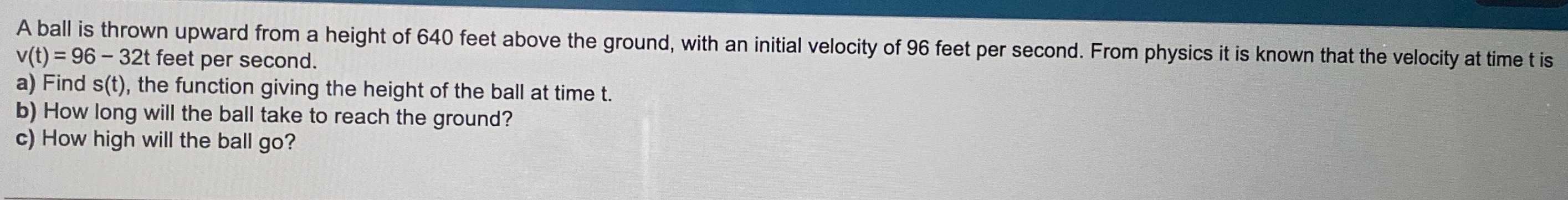### Still have math questions?

Algebra
QuestionA ball is thrown upward from a height of $$640$$ feet above the ground, with an initial velocity of $$96$$ feet per second. From physics it is known that the velocity at time t is $$v ( t ) = 96 - 32 t$$ feet per second.

a) Find $$s ( t )$$ , the function giving the height of the ball at time t.

b) How long will the ball take to reach the ground?

c) How high will the ball go?